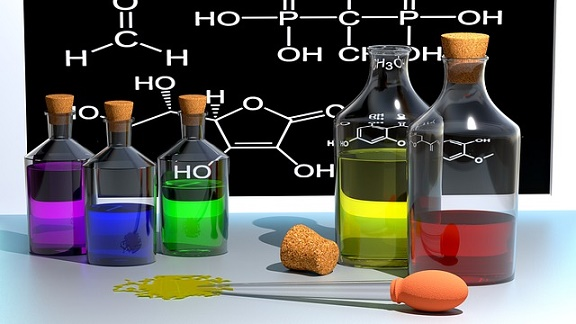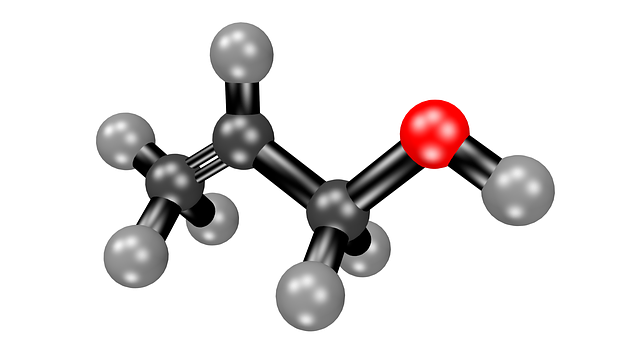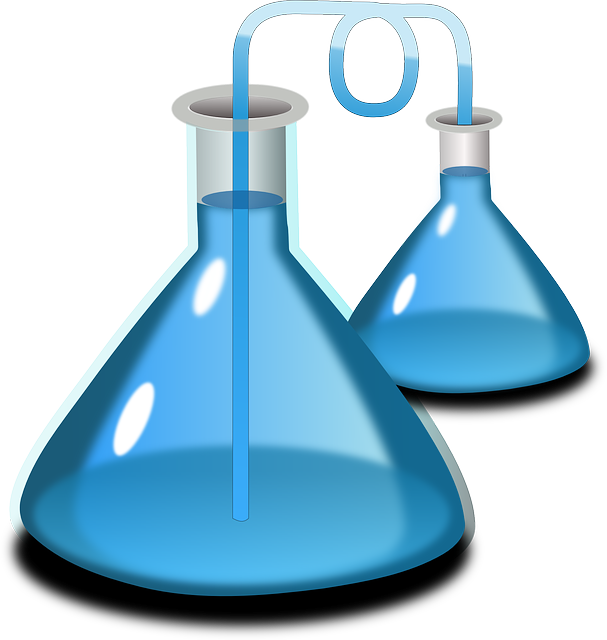# SAT / ACT Prep Online Guides and TipsAre you learning chemistry but don’t quite understand the solubility product constant or want to learn more about it? Not sure how to calculate molar solubility from \$K_s_p\$? The solubility constant, or \$K_s_p\$, is an important part of chemistry, particularly when you’re working with solubility equations or analyzing the solubility of different solutes. When you have a solid grasp of \$K_s_p\$, those questions become much easier to answer!

In this \$K_s_p\$ chemistry guide, we’ll explain the \$K_s_p\$ chemistry definition, how to solve for it (with examples), which factors affect it, and why it’s important. At the bottom of this guide, we also have a table with the \$K_s_p\$ values for a long list of substances to make it easy for you to find solubility constant values.

## What Is \$K_s_p\$?

\$K_s_p\$ is known as the solubility constant or solubility product. It’s the equilibrium constant used for equations when a solid substance is dissolving in a liquid/aqueous solution. As a reminder, a solute (what is being dissolved) is considered soluble if more than 1 gram of it can be completely dissolved in 100 ml of water.

\$K_s_p\$ is used for solutes that are only slightly soluble and don’t completely dissolve in solution. (A solute is insoluble if nothing or nearly nothing of it dissolves in solution.) \$K_s_p\$ represents how much of the solute will dissolve in solution.

The value of \$K_s_p\$ varies depending on the solute. The more soluble a substance is, the higher its \$K_s_p\$ chemistry value. And what are the \$K_s_p\$ units? Actually, it doesn’t have a unit! The \$K_s_p\$ value does not have any units because the molar concentrations of the reactants and products are different for each equation. This would mean the \$K_s_p\$ unit would be different for every problem and would be difficult to solve, so in order to make it simpler, chemists generally drop \$K_s_p\$ units altogether. How nice of them!

## How Do You Calculate \$K_s_p\$?

In this section, we explain how to write out \$K_s_p\$ chemistry expressions and how to solve for the value of \$K_s_p\$. For most chemistry classes, you’ll rarely need to solve for the value of \$K_s_p\$; most of the time you’ll be writing out the expressions or using \$K_s_p\$ values to solve for solubility (which we explain how to do in the “Why Is \$K_s_p\$ Important” section).

### Writing \$K_s_p\$ Expressions

Below is the solubility product equation which is followed by four \$K_s_p\$ chemistry problems so you can see how to write out \$K_s_p\$ expressions.

For the reaction \$A_aB_b\$(s) ⇌ \$aA^b^{+}\$(aq) + \$bB^a^{-}\$ (aq)

The solubility expression is \$K_s_p\$= \$[A^b^{+}]^a\$ \$[B^a^{-}]^b\$

The first equation is known as a dissociation equation, and the second is the balanced \$K_s_p\$ expression.

For these equations:

• A and B represent different ions and solids. In these equations, they are also referred to as "products".
• a and b represent coefficients used to balance the equation
• (aq) and (s) indicate which state the product is in (aqueous or solid, respectively)
• Brackets stand for molar concentration. So [AgCl] represents the molar concentration of AgCl.

In order to write \$K_s_p\$ expressions correctly, you need to have a good knowledge of chemical names, polyatomic ions, and the charges associated with each ion. Also, the key thing to be aware of with these equations is that each concentration (represented by square brackets) is raised to the power of its coefficient in the balanced \$K_s_p\$ expression.

Let’s look at a few examples.

#### Example 1

\$PbBr_2\$(s) ⇌ \$Pb^2^{+}\$ (aq) + \$2Br^{¯}\$ (aq)

\$K_s_p\$= \$[Pb^2^{+}]\$ \$[Br¯]^2\$

In this problem, don’t forget to square the Br in the \$K_s_p\$ equation. You do this because of the coefficient “2” in the dissociation equation.

#### Example 2

CuS(s) ⇌ \$Cu^{+}\$ (aq) + S¯(aq)

\$K_s_p\$= [\$Cu^{+}\$] [S¯]

#### Example 3

\$Ag_2CrO_4\$ (s) ⇌ 2\$Ag^{+}\$ (aq) + \$CrO_4^2^{-}\$ (aq)

\$K_s_p\$= \$[Ag^{+}]^2\$ [\$CrO_4^2\$]

#### Example 4

\$Cu_3\$ \$(PO_4)^2\$ (s) ⇌ \$3Cu^2^{+}\$ (aq) + \$2PO_4^3^{¯}\$ (aq)

\$K_s_p\$ = \$[Cu^2^{+}]^3\$ [\$PO_4^3^¯\$]\$^2\$### Solving for \$K_s_p\$ With Solubility

In order to calculate a value for \$K_s_p\$, you need to have molar solubility values or be able to find them.

Question: Determine the \$K_s_p\$ of AgBr (silver bromide), given that its molar solubility is 5.71 x \$10^{¯}^7\$ moles per liter.

First, we need to write out the two equations.

AgBr(s) ⇌ \$Ag^{+}\$ (aq) + \$Br^{¯}\$ (aq)

\$K_s_p\$ = [\$Ag^{+}\$] [\$Br^{¯}\$]

Now, since in this problem we're solving for an actual value of \$K_s_p\$, we plug in the solubility values we were given:

\$K_s_p\$ = (5.71 x \$10^{¯}^7\$) (5.71 x \$10^{¯}^7\$) = 3.26 x \$10^{¯}^13\$

The value of \$K_s_p\$ is 3.26 x \$10^{¯}^13\$

## What Factors Affect \$K_s_p\$?

In this section, we discuss the main factors that affect the value of the solubility constant.

### Temperature

Most solutes become more soluble in a liquid as the temperature is increased. If you’d like proof, see how well instant coffee mixes in a cup of cold water compared to a cup of hot water. Temperature affects the solubility of both solids and gases but hasn’t been found to have a defined impact on the solubility of liquids.

### Pressure

Pressure can also affect solubility, but only for gases that are in liquids. Henry's law states that the solubility of a gas is directly proportional to the partial pressure of the gas.

Henry’s law is written as p=kc, where

• p is the partial pressure of the gas above the liquid
• k is Henry’s law constant
• c is the concentration of gas in the liquid

Henry’s law shows that, as partial pressure decreases, the concentration of gas in the liquid also decreases, which in turn decreases solubility. So less pressure results in less solubility, and more pressure results in more solubility.

You can see Henry’s law in action if you open up a can of soda. When the can is closed, the gas is under more pressure, and there are lots of bubbles because a lot of the gas is dissolved. When you open the can, the pressure decreases, and, if you leave the soda sitting out long enough, the bubbles will eventually disappear because solubility has decreased and they are no longer dissolved in the liquid (they’ve bubbled out of the drink).

### Molecular Size

Generally, solutes with smaller molecules are more soluble than ones with molecules particles. It’s easier for the solvent to surround smaller molecules, so those molecules can be dissolved faster than larger molecules.## Why Is \$K_s_p\$ Important?

Why does the solubility constant matter? Below are three key times you’ll need to use \$K_s_p\$ chemistry.

### To Find the Solubility of Solutes

Wondering how to calculate molar solubility from \$K_s_p\$? Knowing the value of \$K_s_p\$ allows you to find the solubility of different solutes. Here’s an example: The \$K_s_p\$ value of \$Ag_2SO_4\$ ,silver sulfate, is 1.4×\$10^{–}^5\$. Determine the molar solubility.

First, we need to write out the dissociation equation: \$K_s_p\$=\$ [Ag^{+}]^2\$ \$[SO_4^2]\$

Next, we plug in the \$K_s_p\$ value to create an algebraic expression.

1.4×\$10^{–}^5\$= \$(2x)^2\$ \$(x)\$

1.4×\$10^{–}^5\$= \$4x^3\$

\$x\$=[\$SO_4^2\$]=1.5x\$10^{-}^2\$ M

\$2x\$= [\$Ag^{+}\$]=3.0x\$10^{-}^2\$ M

### To Predict If a Precipitate Will Form in Reactions

When we know the \$K_s_p\$ value of a solute, we can figure out if a precipitate will occur if a solution of its ions is mixed. Below are the two rules that determine the formation of a precipitate.

• Ionic product > \$K_s_p\$ then precipitation will occur
• Ionic product < \$K_s_p\$ then precipitation will not occur

### To Understand the Common Ion Effect

\$K_s_p\$ also is an important part of the common ion effect. The common ion effect states that when two solutions that share a common ion are mixed, the solute with the smaller \$K_s_p\$ value will precipitate first.

For example, say BiOCl and CuCl are added to a solution. Both contain \$Cl^{-}\$ ions. BiOCl’s \$K_s_p\$ value is 1.8×\$10^{–}^31\$ and CuCl’s \$K_s_p\$ value is 1.2×\$10^{–}^6\$. BiOCl has the smaller \$K_s_p\$ value, so it will precipitate before CuCl.

## Solubility Product Constant Table

Below is a chart showing the \$K_s_p\$ values for many common substances. The \$K_s_p\$ values are for when the substances are around 25 degrees Celsius, which is standard. Because the \$K_s_p\$ values are so small, there may be minor differences in their values depending on which source you use. The data in this chart comes from the University of Rhode Island’s Department of Chemistry.

## Conclusion: \$K_s_p\$ Chemistry Guide

What is \$K_s_p\$ in chemistry? The solubility product constant, or \$K_s_p\$, is an important aspect of chemistry when studying solubility of different solutes. \$K_s_p\$ represents how much of the solute will dissolve in solution, and the more soluble a substance is, the higher the chemistry \$K_s_p\$ value.

To calculate the solubility product constant, you’ll first need to write out the dissociation equation and balanced \$K_s_p\$ expression, then plug in the molar concentrations, if you’re given them.

The solubility constant can be affected by temperature, pressure, and molecular size, and it’s important for determining solubility, predicting if a precipitate will form, and understand the common ion effect.

## What's Next?

Inconsolable that you finished learning about the solubility constant? Drown your sorrows in our complete guide to the 11 solubility rules.

Looking for other chemistry guides? Learn how to balance chemical equations here, or read through these six examples of physical and chemical change.

Taking chemistry in high school? We've compiled several great study guides for AP Chem, IB Chemistry, and the NY state Chemistry Regents exam.

Need more help with this topic? Check out Tutorbase!

Our vetted tutor database includes a range of experienced educators who can help you polish an essay for English or explain how derivatives work for Calculus. You can use dozens of filters and search criteria to find the perfect person for your needs.Christine Sarikas

Christine graduated from Michigan State University with degrees in Environmental Biology and Geography and received her Master's from Duke University. In high school she scored in the 99th percentile on the SAT and was named a National Merit Finalist. She has taught English and biology in several countries.

Student and Parent Forum

Our new student and parent forum, at ExpertHub.PrepScholar.com, allow you to interact with your peers and the PrepScholar staff. See how other students and parents are navigating high school, college, and the college admissions process. Ask questions; get answers.